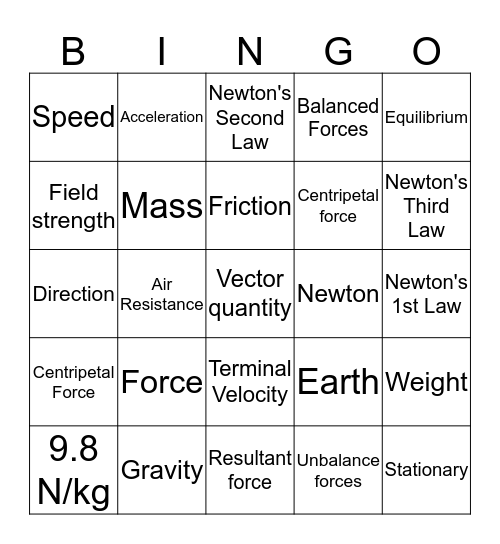ForcesThis bingo card has 25 words: Force, Resultant force, Friction, 9.8 N/kg, Equilibrium, Speed, Field strength, Acceleration, Newton, Terminal Velocity, Centripetal force, Direction, Earth, Weight, Mass, Stationary, Newton's 1st Law, Newton's Second Law, Newton's Third Law, Air Resistance, Vector quantity, Gravity, Centripetal Force, Balanced Forces and Unbalance forces.

Play Online